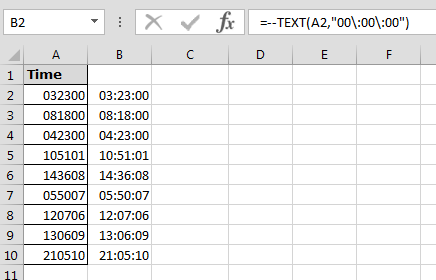# Text to Time Conversion in Microsoft Excel

Original Question:-

How to convert text into time?

I have a text file that I want to import into Excel. I have a column that is
a time field. (Ex. 142200)(hh:mm:ss). When I import it and format it in time,I always get 00:00:00. Is there a command that I need to convert it?

User query: -When we export the text file in Excel file then time format gets changed. Even after applying the time formatting, it is not converting into right time format.Our one of the users has replied: - These numbers will be interpreted as hh:mm:ss
with either opening or importing a text file. 142200, since there's no fractional part (right of the decimal point -- the part that indicates a fraction of a day, or time) will always be zero. This value is a date of 29Apr 2289, at midnight.

=TIME(LEFT(A2,2),MID(A2,3,2), RIGHT(A2,2))
Then this column would be formatted as hh:mm:ss, or according to your desired format.

How to resolve this problem?

Follow below given steps to covert the text in to time:-

• Enter the formula in cell B2.
• =--TEXT(A2,"00\:00\:00")
• Function will return the number.
• Format the cells in to hh:mm:ss
• Copy the same formula in the range.This is the way we can convert the text into time by using the Text function.

Popular Articles:

50 Excel Shortcuts to Increase Your Productivity

How to use the VLOOKUP Function in Excel

How to use the COUNTIF function in Excel

How to use the SUMIF Function in Excel

1.